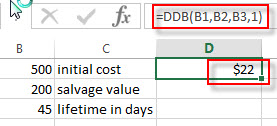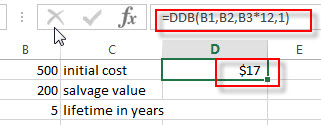# Excel DDB Function

This post will guide you how to use Excel DDB function with syntax and examples in Microsoft excel.

### Description

The Excel DB function returns the depreciation of an asset for a specified period using the double-declining balance method or you can also use some other method to get the depreciation.

The DB function is a build-in function in Microsoft Excel and it is categorized as a Financial Function.

The DB function is available in Excel 2016, Excel 2013, Excel 2010, Excel 2007, Excel 2011 for Mac.

### Syntax

The syntax of the DDB function is as below:

`= DDB(cost, salvage, life, period, [factor])`

Where the DDB function arguments are:

• Cost -This is a required argument. The original cost of the asset.
• Salvage – This is a required argument.  The salvage value at the end of the life of the asset.
• Life – This is a required argument.  The number of periods over which the asset is to be depreciated.
• Period – This is a required argument.  The period in which to calculate the depreciation.
• Factor – This is an optional argument.  The rate at which the balance declines. If the factor argument is omitted, and it will be set the default value as 2.

Note:

• If you do not use the double declining balance method, you can change the factor argument to other value.
• You can use the VDB function if you want to switch to the straight-line depreciation method when depreciation is greater than the declining balance calculation.

### Excel DDB Function Examples

The below examples will show you how to use Excel DB Function to calculate the depreciation of an asset using the double declining balance method.

#1 to get the second day’s depreciation using the double declining balance method, refer to the following formula:

`=DDB(B1,B2,B3,1)`#2 to get the month’s depreciation using the double declining balance method, enter the following formula in Cell C2:

`=DDB(B1,B2,B3*12,1)`### Related Functions

• Excel DB Function
The Excel DB function returns the depreciation of an asset for a specified period using the fixed-declining balance method.The syntax of the DB function is as below:= DB (cost, salvage, life, period, [month])…